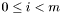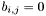#Boost C++ Libraries

...one of the most highly regarded and expertly designed C++ library projects in the world.

This is the documentation for an old version of boost. Click here for the latest Boost documentation.

# boost::numeric::ublas::diagonal_matrix< T, L, A > Class Template Reference

A diagonal matrix of values of type T, which is a specialization of a banded matrix. More...

List of all members.

## Public Types

typedef A::size_type size_type
typedef banded_matrix< T, L, A > matrix_type
typedef A array_type

## Public Member Functions

BOOST_UBLAS_INLINE diagonal_matrix (size_type size)
BOOST_UBLAS_INLINE diagonal_matrix (size_type size, const array_type &data)
BOOST_UBLAS_INLINE diagonal_matrix (size_type size1, size_type size2)
template<class AE >
BOOST_UBLAS_INLINE diagonal_matrix (const matrix_expression< AE > &ae)
BOOST_UBLAS_INLINE
diagonal_matrix
operator= (const diagonal_matrix &m)
template<class AE >
BOOST_UBLAS_INLINE
diagonal_matrix
operator= (const matrix_expression< AE > &ae)

## Detailed Description

### template<class T, class L, class A> class boost::numeric::ublas::diagonal_matrix< T, L, A >

For a-dimensional diagonal matrix,and, ifthen. The default storage for diagonal matrices is packed. Orientation and storage can also be specified. Default is row major unbounded_array.

As a specialization of a banded matrix, the constructor of the diagonal matrix creates a banded matrix with 0 upper and lower diagonals around the main diagonal and the matrix is obviously a square matrix. Operations are optimized based on these 2 assumptions. It is not required by the storage to initialize elements of the matrix.

Template Parameters:
 T the type of object stored in the matrix (like double, float, complex, etc...) L the storage organization. It can be either row_major or column_major. Default is row_major A the type of Storage array. Default is unbounded_array

Generated on Sun Jul 4 20:31:06 2010 for ublas by1.6.1